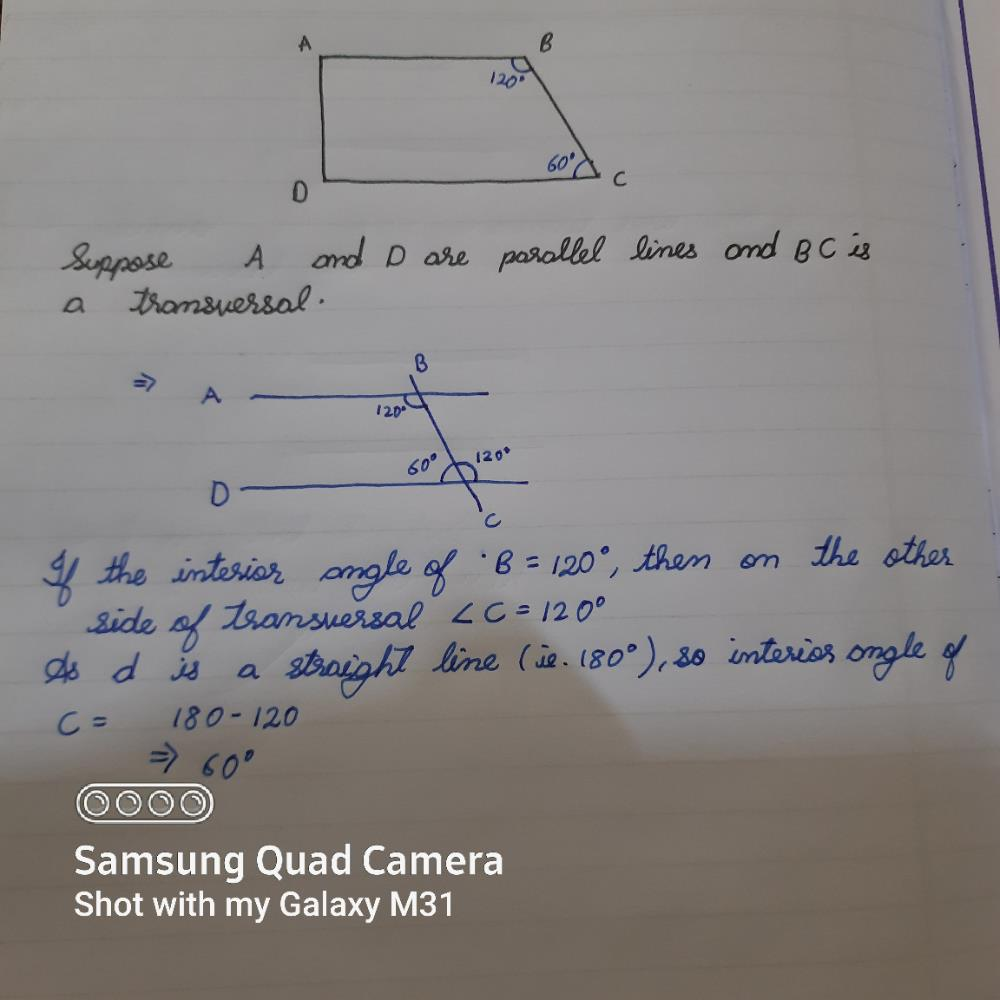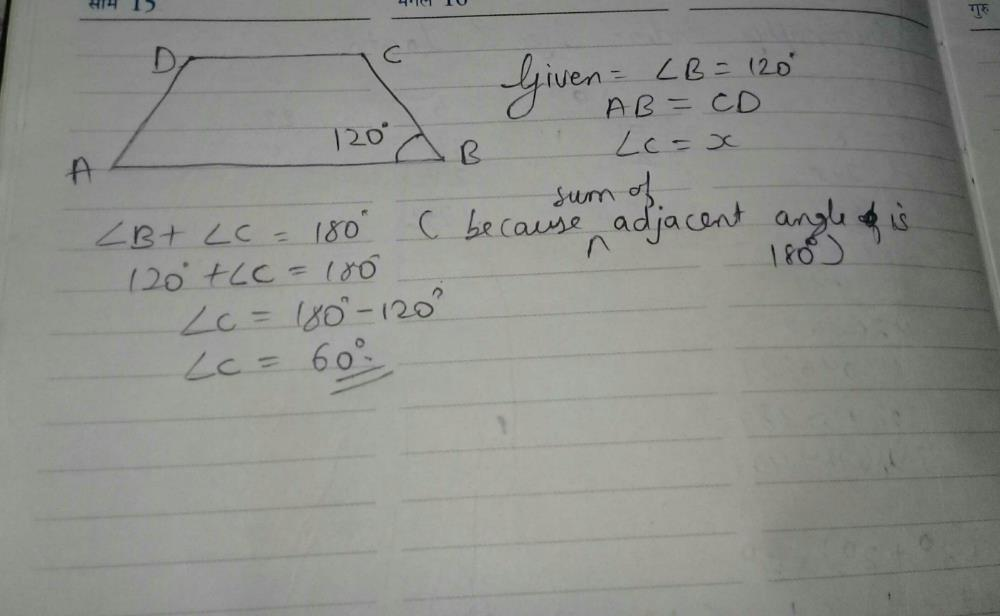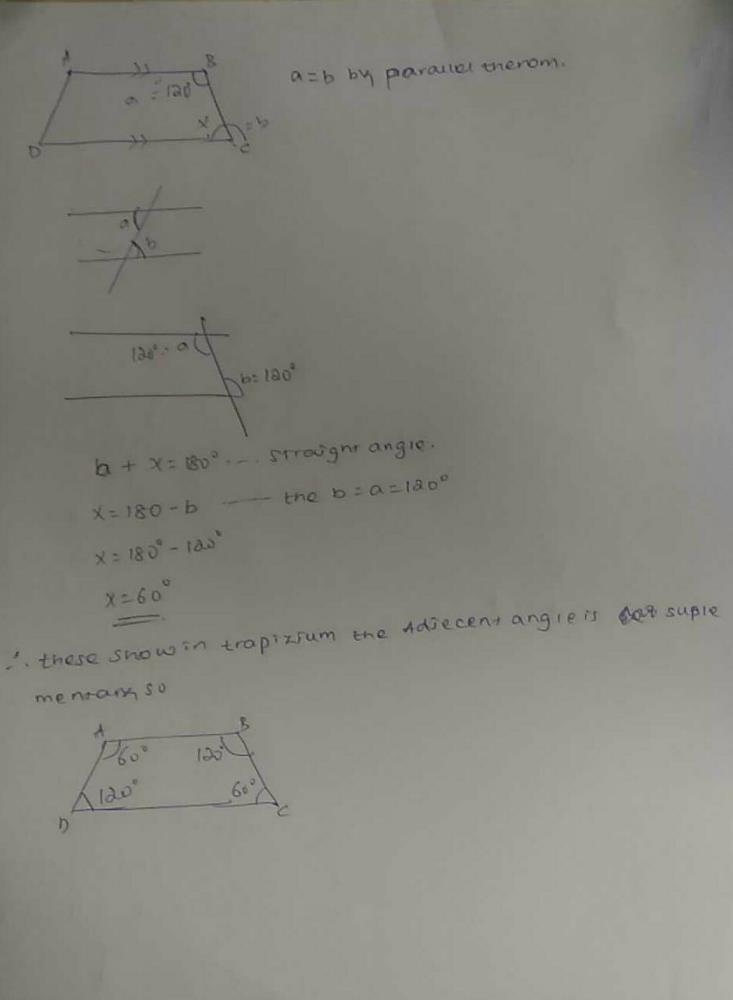Courses

# In a trapezium ABCD angle b is 120degree . find angle c. In which AB is parallel to DC?

## Class 9 Question

By Radhika Gupta · Apr 02, 2020 ·Class 9
Akshat Bhatt answered Apr 02, 2020Sarthak Singh answered Apr 09, 2020Sukhmanpreet Kaur answered 3 days ago
Right answer is x = 60 degree

Suraj Kar answered Apr 09, 2020
60

Kendre Sudarshan answered Apr 26, 2020
Yes

Yogita Sharma answered Apr 12, 2020
60.

Its 60 degree..

Elias Getachew answered 3 weeks agoJaiswal Jaiswal answered 3 days ago
C=60degree

Abhay Kumar answered Apr 12, 2020
given: ABCD is a trapezium and angle B is120*
since, AB||DC and BC is transversal.
then, B+C=180*(co interior angles)
120*+C=180*
C=180*-120*
=60*.
C=60

Ayush Tripathi answered 7 hours ago
A= 90( sum of all interior angle is 360)
B=120
C= 60
D=90 (It is a right angle)
90 +90+ 120+60=360

This discussion on In a trapezium ABCD angle b is 120degree . find angle c. In which AB is parallel to DC? is done on EduRev Study Group by Class 9 Students. The Questions and Answers of In a trapezium ABCD angle b is 120degree . find angle c. In which AB is parallel to DC? are solved by group of students and teacher of Class 9, which is also the largest student community of Class 9. If the answer is not available please wait for a while and a community member will probably answer this soon. You can study other questions, MCQs, videos and tests for Class 9 on EduRev and even discuss your questions like In a trapezium ABCD angle b is 120degree . find angle c. In which AB is parallel to DC? over here on EduRev! Apart from being the largest Class 9 community, EduRev has the largest solved Question bank for Class 9.